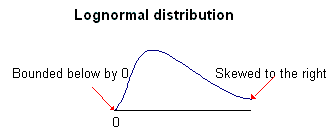### Seeing is believing!

Before you order, simply sign up for a free user account and in seconds you'll be experiencing the best in CFA exam preparation.

##### Subject 9. The Lognormal Distribution
The key properties of the normal distribution have been presented in the LOS above. As a summary, some of the key properties of this distribution are:

• It is symmetrical about the mean.
• It has zero skewness.
• It has a kurtosis of 3.

A random variable, Y, follows a lognormal distribution if its natural logarithm, lnY, is normally distributed. You can think of the term lognormal as "the log is normal." For example, suppose X is a normal random variable, and Y = eX. Therefore, LnY = Ln(eX) = X. Because X is normally distributed, Y follows a lognormal distribution.

• Like the normal distribution, the lognormal distribution is completely described by two parameters: mean and variance.
• Unlike the normal distribution, the lognormal distribution is defined in terms of the parameters of the associated normal distribution. Note that the mean of Y is not equal to the mean of X, and the variance of Y is not equal to the variance of X. In contrast, the normal distribution is defined by its own mean and variance.
• The lognormal distribution is bounded below by 0. In contrast, the normal distribution extends to negative infinity without limit.
• The lognormal distribution is skewed to the right (i.e., it has a long right tail). In contrast, the normal distribution is bell-shaped (i.e., it is symmetrical).The reverse is also true; if a random variable Y follows a lognormal distribution, then its natural logarithm, lnY, is normally distributed.

User Comment
surob Perfect explanation why continuosly compounded return is better than HPY. Good job!!!
achu That is a good explanation of why Cnts compouding is better. I wonder if it's used in the real world, though.
rufi this is a good tool for BSOPM
bahodir what is BSOPM?
bobert Black-Scholes Option Pricing Model
aakash1108 nice.
Seemorr What kind of variable would be lognormally distributed, but not normally?
riouxcf Some variables which have frequent outliers can be made more normal by taking the log. The normal distribution tends to underestimate extremes.
jpducros Is Continuously coumpounding yield always < HPY ?

More generally, can we always write :
Cont Compound Y < HPY < MMY < BEY < EAY

And is BEY = BDiscountYield ?
So many Yields it becomes complicated to remember everything, and the logic behind.
pbielstein You should keep in mind though that in this example the arithmetic average for the discretely compounded returns is taken.

If you take the geometric average then you obtain sqrt{(1-0.2)*(1+0.25)} - 1 = 0
czar Seemor: stock prices (log) and stock returns (normal) as stock prices lowest value can only be 0 while returns can be negative
johntan1979 Shouldn't the discretely compounded quarterly rate of return = (1 + HPR/4)^4 ? 1, not (1 + HPR)^1/4 - 1?
johntan1979 How can the quarterly compounding be less than the annual HPR?
fanDango The quarterly compounding rate is not 4*X = annual HPR because you are compounding the principle and interest each quarter.
Streberli @riouxcf lognormal distributions can't go negative they are used in stockprices not returns. what you mean is student-t distribution with this one you can include the fat tails in of return distributions